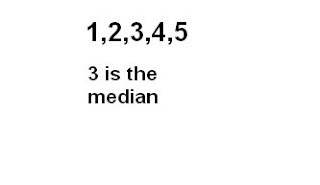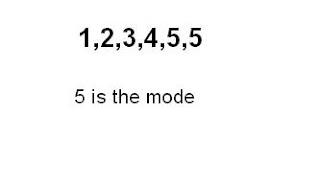### Sean's Measuresof Central Tendancy

Monday, September 29, 2008

Mean- Arrange the data in ascending order. Add them up and divide them by the number of data there is.Median- Arrange data in ascending order. Find the middle number by crossing the numbers from the left to right. If there is two medians, add them and divide them by 2.Mode- Arrange the data in ascending numerical order. Find the most common number.Range- Subtract the biggest number and the smallest number in the set of data.HERE IS A VIDEO THAT EXPLAINS MMM: